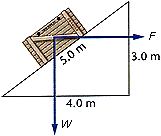# How much work done by friction? (coefficient given)

• Lothar
In summary, the crate is pushed up an inclined plane by a worker, and the coefficient of friction is 0.20. The force of friction is what does the work, and the crate is pushed at a constant horizontal speed.

#### Lothar

Hello everyone,
I've been working on this problem for the last hour and a half. My teacher has asked us to calculate the work done by friction on an inclined plane. This is the problem:
A worker pushes a crate weighing W = 95 N up an inclined plane. The worker pushes the crate horizontally, parallel to the ground, as illustrated in Figure 10-21.(c) The coefficient of friction is µ = 0.20. How much work is done by friction? (Be careful with the signs you use.)

How do I do this? We have not learned anything about coefficient of friction in my class.

Thank you

I don't know why you continue in this thread, since I answered you in the other thread...

Friction = coefficient of friction * Normal force

So I would say, calculate the normal force, use the given coefficient of friction and there you have it. W=Fs, so multiply by s to find the work done by the force of friction.

Hi Lothar,

You first need to calculate the force exerted due to friction, the equation for this can be found here:

http://en.wikipedia.org/wiki/Friction

Have you been supplied with a distance that the crate is pushed?

@ ImAnEngineer
I understand that that is the formula. I don't know how to use it though. And I moved this to a new topic because I new that people would back away from a long topic (my last one), and if they did click it, they would not spend the time reading all the way to my new question.

@ astrorob
No I was not supplied with a distance. I put all information known in the first post.
I do have the amount of work done by friction though, as well as the amount of work done if a man pushes at a certain force.

astrorob said:
Hi Lothar,
Have you been supplied with a distance that the crate is pushed?
It's in the figure; 5m.

Lothar said:
@ ImAnEngineer
I understand that that is the formula. I don't know how to use it though.
How could you not understand how to use it? Just fill it in... Or do you not know how to find the normal force? In that case, how about drawing the resultant vector of F and W first?